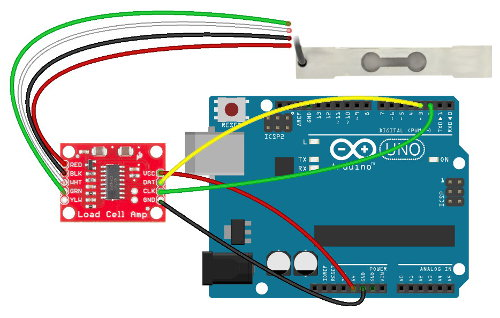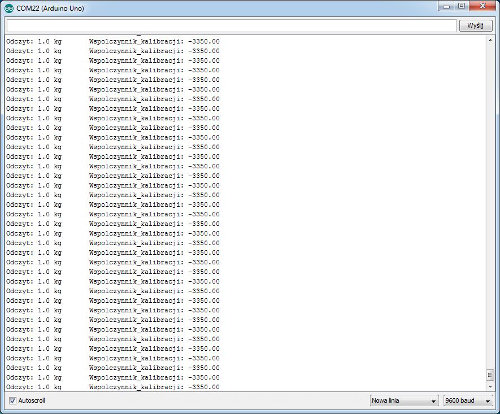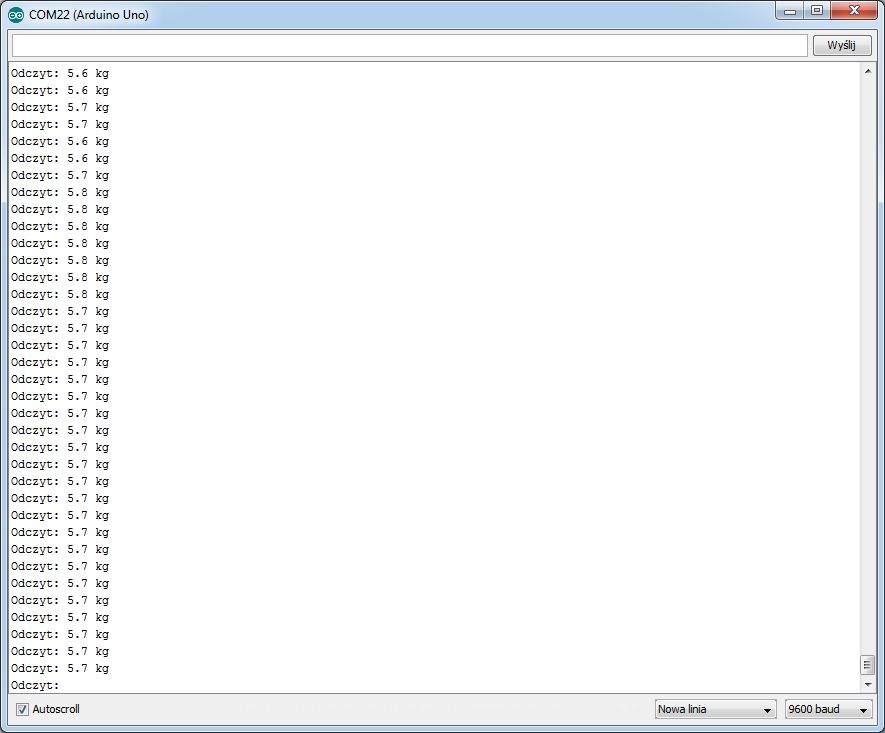# Use a strain gauge beam with Arduino

## Use beams tensometrycznej using Arduino

Beam tensometryczna allows you to weigh the item. This short guide shows how to handle it using the Arduino Uno.

### Connection

The module works directly with the Arduino, so no need for an external source of energy. Contacts connect your null-modem according to the following table:

Pin of the amplifierPin Arduino
VCC5 V
DATE3
CLK2
GNDGND

On the other side of the amplifier module connect your null modem beam tensometryczną on the colors of the wires.

• Red - red
• BLK - black
• WHT - white
• GRN - greenWiring diagram for beams, the amplifier and the Arduino Uno.

#### Service

At the beginning of the library you want to add to the environment of the Arduino (Sketch -> Include Library -> Add .ZIP Library...).

The example programs have been used from the included library. First you need to run the calibration program SparkFun_HX711_Calibration (File -> Examples -> HX711 -> SparkFun_HX711_Calibration). Thanks to him, we can determine the ratio used for beam tensometrycznej.

Run the system with no load on the beam. Then we put the object with known mass. Now with the buttons "+" and "-" (or "a" and "C") install the reading so that it agrees with our known mass, for example,The screen of the monitor the serial port.

Below is the program (based on libraries)

```#include "HX711.h"

#define DOUT 3 //pin 3 Arduino and the sensor output of DATES
#define CLK 2 //Arduino pin 2 and the output CLK of the sensor

HX711 scale(DOUT, CLK);

float calibration_factor = -7050; //calibration factor

void setup() {
Serial.begin(9600);
Serial.println("HX711 program calibration");
Serial.println("Remove the load from the beam");
Serial.println("After enabling reading while holding a known weight on the beam");
Serial.println("Press + or-to increase wspolczynnik calibration");
Serial.println("Give - or to increase wspolczynnik calibration");

scale.set_scale();
scale.tare(); //Resets the scale to 0

Serial.print("Zero factor "); //Can be used in order to remove the need for calibration of the scale. It is useful in projects with a fixed Scully
Serial.println(zero_factor);
}

void loop() {

scale.set_scale(calibration_factor); //Alignment calibration factor

Serial.print(scale.get_units(), 1);
Serial.print(" kg"); //we can Freely choose kilograms or pounds, because between them there is a linear relationship
Serial.print(" Wspolczynnik_kalibracji: ");
Serial.print(calibration_factor);
Serial.println();

if(Serial.available())
{
char temp = Serial.read(); //load entered value and the change in calibration factor
if(temp == '+' || temp == 'a')
calibration_factor += 10;
else if(temp == '-' || temp == 'C')
calibration_factor -= 10;
}
}
```

Then the value read should be used in the program SparkFun_HX711_Example (File -> Examples -> HX711 -> SparkFun_HX711_Example). We use spolszczonego program:

```#include "HX711.h"

#define calibration_factor -3350.0 //Read the value from the calibration program

#define DOUT 3 //pin 3 Arduino and the sensor output of DATES
#define CLK 2 //Arduino pin 2 and the output CLK of the sensor

HX711 scale(DOUT, CLK);

void setup() {
Serial.begin(9600);
Serial.println("HX711 scale demo");

scale.set_scale(calibration_factor); //Parameter calibration
scale.tare(); //Resetting at the beginning

}

void loop() {
Serial.print(scale.get_units(), 1); //scale.get_units() returns a float
Serial.print(" kg"); //we can Freely choose kilograms or lbs, but when you change to a change in the calibration factor
Serial.println();
}
```

Now we can weigh any items. The result of the program shown in the screenshot below:The screen of the monitor the serial port.